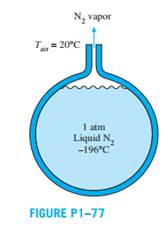Create an Account

Home / Questions / Using EES or other software plot the rate of evaporation of liquid nitrogen as a function ...

Using EES or other software plot the rate of evaporation of liquid nitrogen as a function of the ambient air temperature in the range of 0°C to 40°C Discuss the results Problem 1 77 The boiling

Using EES (or other) software, plot the rate of evaporation of liquid nitrogen as a function of the ambient air temperature in the range of 0°C to 40°C. Discuss the results.

Problem 1–77

The boiling temperature of nitrogen at atmospheric pressure at sea level (1 atm) is 2196°C. Therefore, nitrogen is commonly used in low temperature scientific studies since the temperature of liquid nitrogen in a tank open to the atmosphere remains constant at 2196°C until the liquid nitrogen in the tank is depleted. Any heat transfer to the tank results in the evaporation of some liquid nitrogen, which has a heat of vaporization of 198 kJ/kg and a density of 810 kg/m3 at 1 atm. Consider a 4-m-diameter spherical tank initially filled with liquid nitrogen at 1 atm and 2196°C. The tank is exposed to 20°C ambient air with a heat transfer coefficient of 25 W/m2 ·K. The temperature of the thin-shelled spherical tank is observed to be almost the same as the temperature of the nitrogen inside. Disregarding any radiation heat exchange, determine the rate of evaporation of the liquid nitrogen in the tank as a result of the heat transfer from the ambient air.Jul 22 2020 View more View LessSubscribe To Get Solution